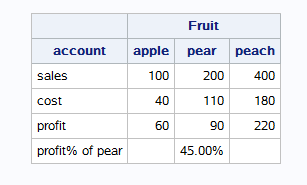## proc report - calculated value

Hi there,

I am new to proc report procedures, and I would like to ask a question regarding calculated value, and using calculated value to generate another calculated value.

For example, using the sample data below: (Fruit is spanned header)

Fruit
account    apple     pear    peach
sales           100       200       400
cost            40          110         180

I'd like to create a report using SAS proc report. Specifically, I'd like to calculate the profit, and profit % for pear only. The output should look like the following:

Fruit
account      apple     pear    peach
sales             100       200      400
cost                40        110         180
profit              60        90         220
profit% of pear         45%

Both profit and profit% of pear are calculated values. Could you please teach me how to do it using proc report?
Thank you.Ksharp
Super User

## Re: proc report - calculated value

``````/*
It is not right tool to calculate a variable,
*/

data have;
input account : \$20.  apple     pear    peach;
id=1;
cards;
sales           100       200       400
cost            40          110         180
;
run;

options missing=' ';
proc report data=have nowd;
columns id account ('Fruit' apple     pear    peach);
define id/order noprint;
define account/display;
define apple/analysis sum;
define pear/analysis sum;
define peach/analysis sum;

compute peach;
if account='sales' then do;apple_s=apple.sum;pear_s=pear.sum;peach_s=peach.sum;end;
if account='cost'  then do;apple_c=apple.sum;pear_c=pear.sum;peach_c=peach.sum;end;
endcomp;
compute after id;
account='profit';
apple.sum=apple_s-apple_c;
pear.sum=pear_s-pear_c;
peach.sum=peach_s-peach_c;
endcomp;
compute after;
account='profit% of pear';
apple.sum=.;
pear.sum=(pear_s-pear_c)/pear_s ;
peach.sum=.;
call define('pear.sum','format','percentn8.2');
endcomp;
break after id/summarize;
rbreak after/summarize;
run;``````Discussion stats